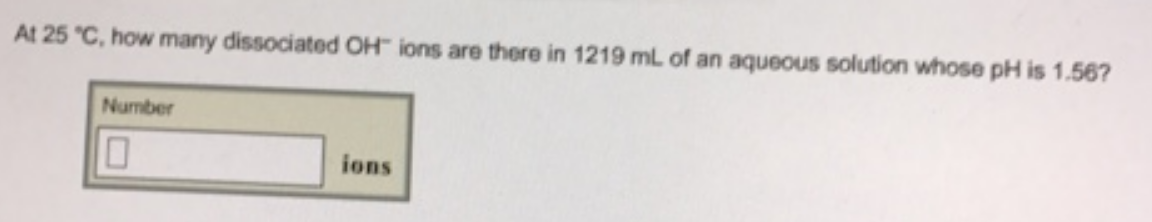Chemistry Practice Problems pH and pOH Practice Problems Solution: At 25 C, how many dissociated OH ions are there in...

# Solution: At 25 C, how many dissociated OH ions are there in 1219 mL of an aqueous solution whose pH is 1.56?

###### Problem

At 25 C, how many dissociated OH ions are there in 1219 mL of an aqueous solution whose pH is 1.56?View Complete Written Solution

pH and pOH

pH and pOH

#### Q.  Calculate the poH of a 0.0321 M HCIO4 solution at the following temperatures

Solved • Fri May 04 2018 18:34:41 GMT-0400 (EDT)

pH and pOH

#### Q. 1. Three solutions have the following pH:      pH = 4.3      pH = 6.8      pH = 11.5      a) Which solution contains the highest H3O+ ion concentratio...

Solved • Fri May 04 2018 13:14:02 GMT-0400 (EDT)

pH and pOH

#### Q. What are the concentrations of hydrogen ion and hydroxide ion in household ammonia, an aqueous solution of NH3 that has a pH of 12.3?

Solved • Tue May 01 2018 17:23:50 GMT-0400 (EDT)

pH and pOH

#### Q. 0.90 g of sodium hydroxide (NaOH) pellets are dissolved in water to this solution? Express the pH numerically to two decimal places. pH = 11.75 What i...

Solved • Sun Apr 29 2018 13:08:04 GMT-0400 (EDT)